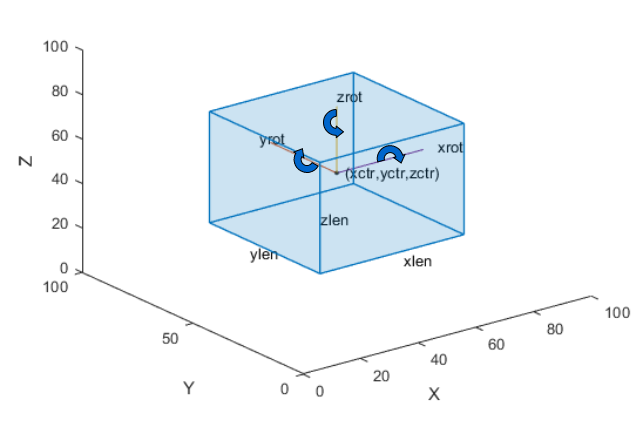# bboxwarp

Apply geometric transformation to bounding boxes

## Syntax

``bboxB = bboxwarp(bboxA,tform,ref)``
``[bboxB,indices] = bboxwarp(bboxA,tform,ref)``
``[___] = bboxwarp(___,'OverlapThreshold',threshold)``

## Description

example

````bboxB = bboxwarp(bboxA,tform,ref)` transforms bounding boxes in `bboxA` according to the geometric transformation defined by `tform`. Bounding boxes can be axis-aligned rectangles, rotated rectangles, or cuboids. The spatial reference object, `ref`, defines the output view into which the boxes are transformed.```
````[bboxB,indices] = bboxwarp(bboxA,tform,ref)` additionally returns a vector of indices that indicate which bounding boxes in `bboxA` correspond to the warped versions in the output, `bboxB`.```
````[___] = bboxwarp(___,'OverlapThreshold',threshold)` additionally sets an overlap threshold.```

## Examples

collapse all

`I = imread('peppers.png');`

Define bounding boxes and labels.

```bboxA = [ 410 230 100 90 186 78 80 60 ]```
```bboxA = 2×4 410 230 100 90 186 78 80 60 ```
```labelsA = [ "garlic" "onion" ];```

Define a transform to horizontally flip and translate the image.

`tform = affine2d([-1 0 0; 0 1 0; 50 50 1]);`

Create an output view for `imwarp`.

`rout = affineOutputView(size(I),tform);`

Warp the image.

`J = imwarp(I,tform,'OutputView',rout);`

Warp the boxes.

```[bboxB,indices] = bboxwarp(bboxA,tform,rout); labelsB = labelsA(indices);```

Display the results.

```annotatedI = insertObjectAnnotation(I,'Rectangle',bboxA,labelsA); annotatedJ = insertObjectAnnotation(J,'Rectangle',bboxB,labelsB); figure montage({annotatedI, annotatedJ})```## Input Arguments

collapse all

Bounding boxes, specified as an M-by-4, M-by-5, or M-by-9 nonsparse numeric matrix of M bounding boxes. Each row, M, of the matrix defines a bounding box as either an axis-aligned rectangle, a rotate rectangle, or a cuboid. The table below describes the format of the bounding boxes.

Bounding BoxDescription
Axis-aligned rectangle

Defined in pixel coordinates as an M-by-4 numeric matrix with rows of the form [x y w h], where:

• M is the number of axis-aligned rectangles.

• x and `y` specify the upper-left corner of the rectangle.

• w specifies the width of the rectangle, which is its length along the x-axis.

• h specifies the height of the rectangle, which is its length along the y-axis.

Rotated rectangle

Defined in spatial coordinates as an M-by-5 numeric matrix with rows of the form [xctr yctr xlen ylen yaw], where:

• M is the number of rotated rectangles.

• xctr and yctr specify the center of the rectangle.

• xlen specifies the width of the rectangle, which is its length along the x-axis before rotation.

• ylen specifies the height of the rectangle, which is its length along the y-axis before rotation.

• yaw specifies the rotation angle in degrees. The rotation is clockwise-positive around the center of the bounding box.Cuboid

Defined in spatial coordinates as an M-by-9 numeric matrix with rows of the form [xctr yctr zctr xlen ylen zlen xrot yrot zrot], where:

• M is the number of cuboids.

• xctr, yctr, and zctr specify the center of the cuboid.

• xlen, ylen, and zlen specify the length of the cuboid along the x-axis, y-axis, and z-axis, respectively, before rotation.

• xrot, yrot, and zrot specify the rotation angles of the cuboid around the x-axis, y-axis, and z-axis, respectively. The xrot, yrot, and zrot rotation angles are in degrees about the cuboid center. Each rotation is clockwise-positive with respect to the positive direction of the associated spatial axis. The function computes rotation matrices assuming `ZYX` order Euler angles [xrot yrot zrot].

The figure shows how these values determine the position of a cuboid.Geometric transformation, specified in an `affine2d` object. bboxwarp function supports only scale, rotation, and translation affine transformations.

Spatial reference, specified as an `imref2d` object returned by the `imwarp` or `affineOutputView` function. The object defines the output view to transform boxes. Boxes that are transformed completely outside of the output view defined by `ref` are discarded.

```[J,rout] = imwarp(I,tform); [bboxB,indices] = bboxwarp(bboxA,tform,rout); ```
```rout = affineOutputView(size(I),tform) J = imwarp(I,tform,'OutputView',rout); [bboxB,indices] = bboxwarp(bboxA,tform,rout); ```

Overlap threshold, specified as a positive scalar less than or equal to `1`. The amount of overlap between transformed boxes and the area defined by the output view is defined as:

area(bbox intersect bounding rectangle) / area(bbox)

If the computed overlap value is greater than the value of the `threshold` property, then the transformed boxes are clipped to the bounding rectangle border. Otherwise, the boxes are discarded. Lowering the threshold can result in parts of the object getting discarded.

## Output Arguments

collapse all

Warped bounding boxes, returned as an M2-by-N matrix of M2 bounding boxes. The number of bounding boxes returned is less than the number of bounding boxes in the input. Each row, M2, of the matrix defines one bounding box of the same type as the input `bboxA`.

Indices, returned as a vector of integers. The indices indicate which bounding boxes in the input, `bboxA`, that correspond to the warped versions in the output, `bboxB`.